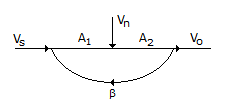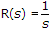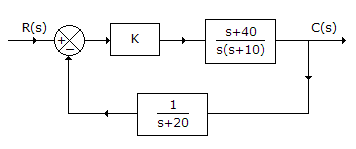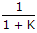# Electronics and Communication Engineering - Automatic Control Systems

### Exercise :: Automatic Control Systems - Section 6

31.

For a second order system delay time td is the time required to reach

 A. half the final value for the very first time B. the final value for the very first time C. 90% of the final value for the very first time D. none of the above

Explanation:

No answer description available for this question. Let us discuss.

32.

Coulomb friction is

 A. the force of sliding friction between dry surfaces B. the force of friction between moving surfaces separated by viscous fluid C. the force required to initiate motion between two contacting surfaces D. all of the above

Explanation:

No answer description available for this question. Let us discuss.

33.

A feedback amplifier has forward gains A1, A2 and feedback factor β as shown in signal flow graph of the given figure. Because of feedback as shown in signal noise ratio at output willA. increase B. decrease C. be unaffected D. depend on value of β

Explanation:

No answer description available for this question. Let us discuss.

34.

For the system in the given figure. Then steady state error isA.B. K C. Zero D. 1

Explanation:

No answer description available for this question. Let us discuss.

35.

The damping ratio of a pair of complex poles when expressed in terms of angle θ measured from jω axis equal to

 A. sin θ B. cos θ C. tan θ D. cot θ